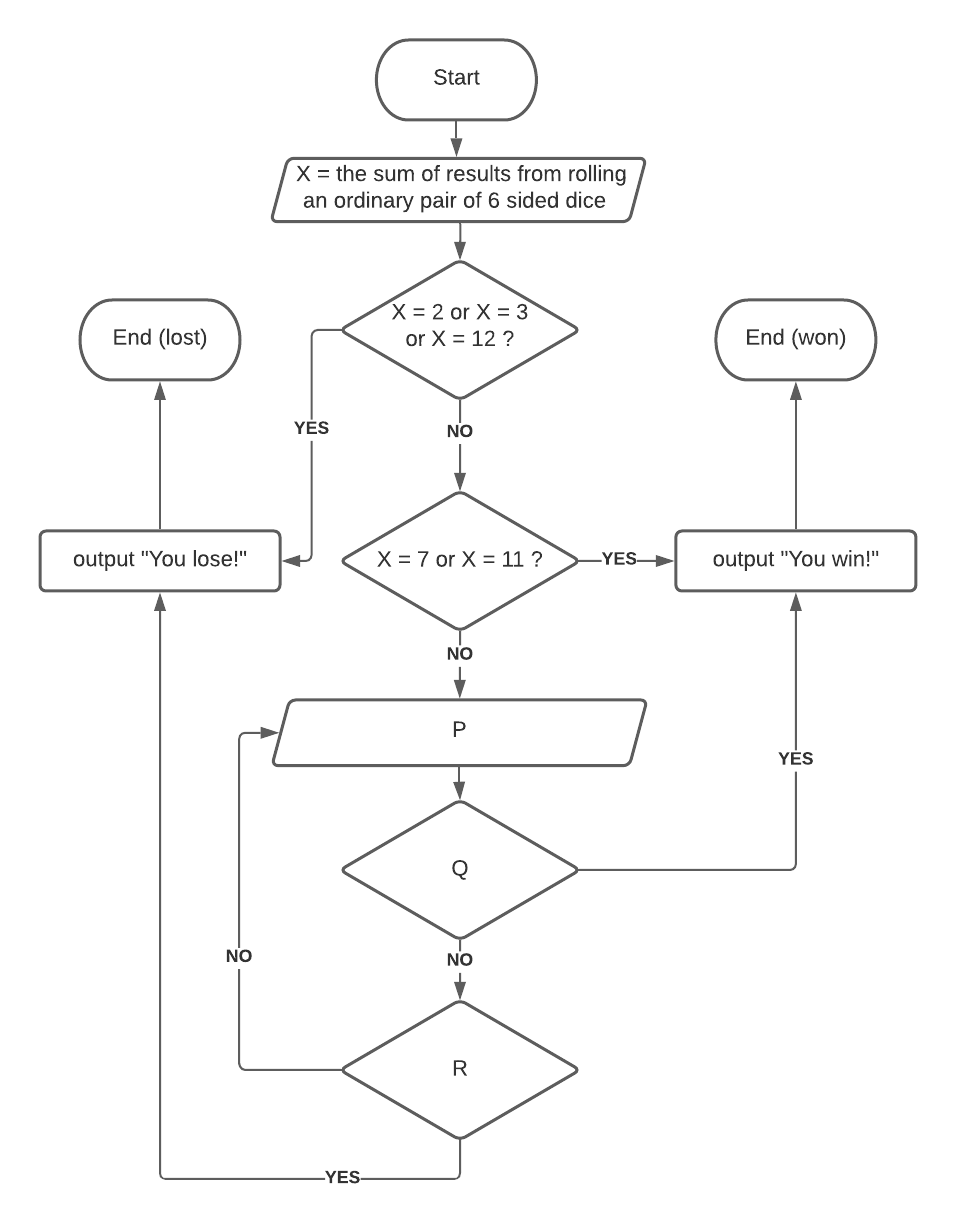Login or Create an Account to view the mark scheme, comment, and add to a test
Consider a game where two dice are rolled and the sum calculated. If a player rolls a sum of 2, 3, or 12 on the first roll, that player loses. If a player rolls a sum of 7 or 11 on the first roll, that player wins. Any other first roll sum becomes the player's new target to win. The player must keep rolling until they either roll their new target to win or a 7 to lose. For example, if a player rolls a 10 on the first roll, their target sum to win becomes 10 and they must keep rolling until they either roll a 10 to win or a 7 to lose.(a).
In order to complete the above flow chart representation of the game logic described, express appropriate logic for steps P, Q & R.

(b).
Given a function called getRandomRoll() which returns a random integer between 1 and 6 inclusive, complete the following pseudocode function called rollTwoDice() by having it return the sum of results from a simulated rolling of a pair of ordinary 6 sided dice.

function rollTwoDice() // insert pseudocode here end function
(c).
Complete the following pseudocode function called playGame() which implements the full game logic illustrated in the above flow chart. Assume the rollTwoDice() function is working as specified previously.

function playGame() X = rollTwoDice() // insert code here end function

HomeThe Right Test - Fast